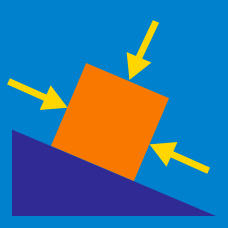Classical Mechanics

# Action-reaction pairs on free body diagrams

Out camping, you decide to hang your food off the ground to keep it away from raccoons and other small scavengers. There are two very tall trees on your campsite $15~\mbox{meters}$ apart and you need to hang $20~\mbox{kg}$ of food exactly between them so the food remains at least $1~\mbox{m}$ off the ground. You head out to the local camping store where they sell $20~\mbox{m}$ long cords that have different maximum tensions they can provide without breaking. The higher the maximum tension, the more expensive the cord. So you can buy the cheapest appropriate cord, what is the minimum tension in Newtons necessary to support the food?

Details and assumptions

• The acceleration of gravity is $-9.8~\mbox{m/s}^2$.
• The cords are effectively massless.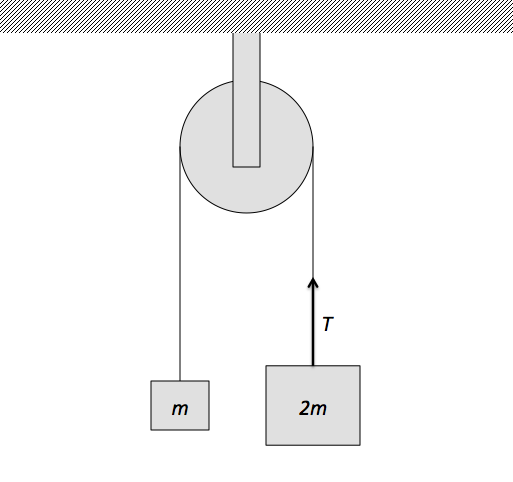Two masses $m$ and $2m$ are attached on each side of a frictionless fixed pulley. If a man who was holding the mass $2m$ releases it, what is the reaction force of the tension in the string $T$ exerted on the mass $2m?$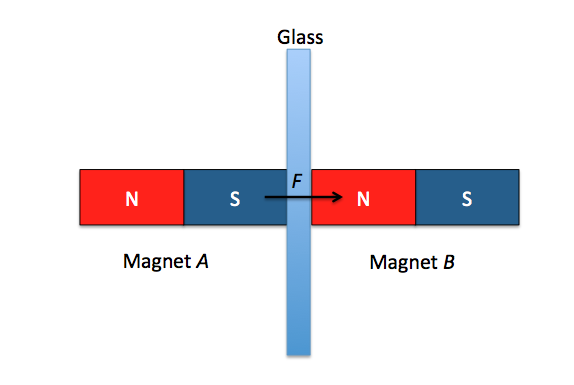Magnet $A$ and magnet $B$ are laid as shown in the above diagram, with a glass in between them. Find the reaction force of the magnetic attraction $F$ that is exerted on $A.$

Assume that there are no magnetic objects other than magnets $A$ and $B.$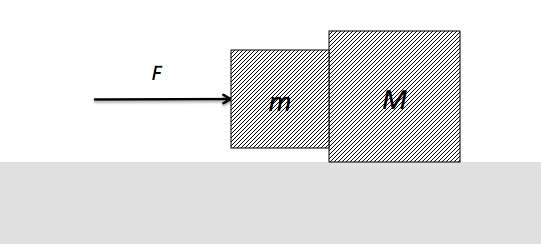A block of mass $m$ is held in contact with a block of mass $M$ by applying a horizontal force of $F$ on it, as shown in the figure above. The block of mass $M$ is lying on a horizontal frictionless surface. The coefficient of static friction between the two blocks is $\mu.$ Which of the following forces acting on the block of mass $m$ has a reaction force?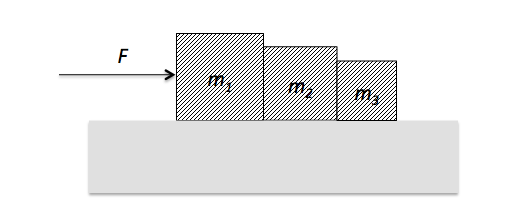Three blocks of respective masses $m_1, m_2 \text{ and } m_3$ are placed in contact on a horizontal frictionless surface. If we apply a force of $F$ by hand to $m_1,$ what is the reaction pair of the force $F?$

×